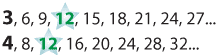Subtraction of fractions with different and unrelated denominators

To subtract fractions with different denominators we first convert to equivalent fractions with the same denominator.

\begin{align}\frac{3}{4} − \frac{2}{3} &= \frac{9}{12} − \frac{8}{12} \text{(convert to equivalent fraction with the same denominator)} \\&= \frac{1}{12} \end{align}

Both 3 and 4 are factors of 12 and 12 is the Lowest Common Multiple (LCM) of 3 and 4 as we can see in the list of multiples of 3 and 4 below:Detailed description